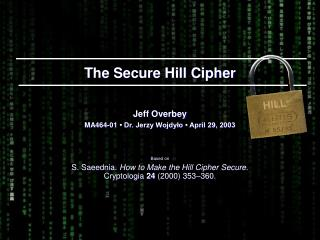DownloadDownload PresentationThe Secure Hill Cipher

# The Secure Hill Cipher

Download Presentation## The Secure Hill Cipher

- - - - - - - - - - - - - - - - - - - - - - - - - - - E N D - - - - - - - - - - - - - - - - - - - - - - - - - - -
##### Presentation Transcript

1. The Secure Hill Cipher HILL Jeff Overbey MA464-01 • Dr. Jerzy Wojdyło • April 29, 2003 Based on S. Saeednia. How to Make the Hill Cipher Secure.Cryptologia 24 (2000) 353–360.

2. The Scenario

3. The Scenario Alice

4. The Scenario Bob

5. The Scenario Oscar

6. The Scenario

7. Sender Recipient Nosy Neighbor The Scenario Insecure Channel

8. Beforehand… Alice and Bob privately share matrix K, invertible over Zm To encrypt a matrix X over Zm… Compute Y = KX Send Y To decrypt Y… Compute X = K–1Y Hill Cipher

9. Sender Recipient Nosy Neighbor Why the Hill Cipher Isn’t Secure Insecure Channel

10. Sender Recipient Nosy Neighbor Why the Hill Cipher Isn’t Secure “Hey Bob,wassup?” Insecure Channel

11. Sender Recipient Nosy Neighbor Why the Hill Cipher Isn’t Secure Insecure Channel

12. Sender Recipient Nosy Neighbor Why the Hill Cipher Isn’t Secure Insecure Channel

13. Sender Recipient Nosy Neighbor Why the Hill Cipher Isn’t Secure Insecure Channel Y = KX

14. Sender Recipient Nosy Neighbor Why the Hill Cipher Isn’t Secure Insecure Channel

15. Sender Recipient Nosy Neighbor Why the Hill Cipher Isn’t Secure Insecure Channel HEYBOBWAS Known Plaintext

16. Sender Recipient Nosy Neighbor Why the Hill Cipher Isn’t Secure Insecure Channel HEYBOBWAS Known Plaintext

17. KNOWNPLAINTEXTATTACK KNOWNPLAINTEXTATTACK Sender Recipient hahahaHAHAHAHA… Nosy Neighbor Why the Hill Cipher Isn’t Secure Insecure Channel HEY BOB,WASSUP?

18. Beforehand… Alice and Bob privately share matrix K, invertible over Zm To encrypt a matrix X over Zm… Compute Y = KX Send Y To decrypt Y… Compute X = K–1Y Secure Hill Cipher Beforehand… • Alice and Bob privately share matrix K, invertible over Zm To encrypt a matrix X over Zm… • Choose a vector t over Zm • Form permutation matrix Pt • Compute Kt = Pt–1KPt • Compute Y = KtX and u = Kt • Send (Y,u) To decrypt (Y,u)… • Compute t = K–1u • Compute Pt • Compute Kt–1 = Pt–1K–1Pt • Compute X = Kt–1Y Note that (Kt)–1 = (K–1)t Hill Cipher

19. Permutation Matrices I = P23 =

20. Permutation Matrices I = P23 = A permutation matrix is a matrix with exactly one 1 in each row and column and zeros elsewhere. P12,23 =

21. Permutation Matrices

22. Vector Representation P23 = P12,23 =

23. Beforehand… Alice and Bob privately share matrix K, invertible over Zm To encrypt a matrix X over Zm… Compute Y = KX Send Y To decrypt Y… Compute X = K–1Y Hill Cipher Secure Hill Cipher Beforehand… • Alice and Bob privately share matrix K, invertible over Zm To encrypt a matrix X over Zm… • Choose a vector t over Zm • Form permutation matrix Pt • Compute Kt = Pt–1KPt • Compute Y = KtX and u = Kt • Send (Y,u) To decrypt (Y,u)… • Compute t = K–1u • Compute Pt • Compute Kt–1 = Pt–1K–1Pt • Compute X = Kt–1Y

24. The Easy Way to Encrypt • Require t to be the vector representation of a permutation matrix • N.B.: This is for example only—it is not practical “in the field.”

25. An Example “Hey Bob, wassup?”

26. Form permutation matrix Pt: An Example Choose a permutation vector: 3 rows, since K is 3×3

27. Compute Kt = Pt–1KPt : An Example Find Pt–1:

28. An Example Find Pt–1: Compute Kt = Pt–1KPt : Permutation of K

29. An Example Compute Y = KtX: Compute u = Kt:

30. An Example Compute Y = KtX: Compute u = Kt:

31. An Example Send (Y,u) to Bob

32. Sender Recipient Nosy Neighbor The Scenario

33. Sender Recipient Nosy Neighbor The Scenario

34. Sender Recipient Nosy Neighbor The Scenario

35. Sender Recipient Nosy Neighbor The Scenario

37. Compute t = K–1u and derive Pt: An Example Compute K–1:

38. Compute X = Kt–1Y: An Example Compute Kt–1 = Pt–1K–1Pt:

39. Compute X = Kt–1Y: An Example Compute Kt–1 = Pt–1K–1Pt: “Hey Bob, wassup?”

40. KNOWNPLAINTEXTATTACK KNOWNPLAINTEXTATTACK An Example But that was just a regular Hill cipher with a fancy key…

41. The Actual Really Secure Hill Cipher HILL Jeff Overbey MA464-01 • Dr. Jerzy Wojdyło • April 29, 2003 Based on S. Saeednia. How to Make the Hill Cipher Secure.Cryptologia 24 (2000) 353–360.

42. The Actual Really Secure Hill Cipher HILL or How to Secure the Secure Hill Cipher

43. Ensuring Security • X should have two columns • Why? Suppose K is n × n and X is n × s. • If s n… • If 2 < s< n… • If s = 2… • Pt should be different for each encryption • Theoretically, this can be ensured by choosing a different t for each encryption • We did this by requiring t to be a vector representation of a permutation matrix, but this is not the best solution.

44. The  Function Let n denote the set of n × n permutation matrices. : Zmn  n Ideally, •  is onto •  is 1-1

45. y = 26n y = n! The  Function Let n denote the set of n × n permutation matrices. : Zmn  n Is it possible? • Zmn = mn • n = n! • Zmn n An “ideal” function DNE.

46. The  Function Ideas? Awkward silence…

47. Apologies for the horrible background image pun.

48. Apologies for the horrible background image pun.